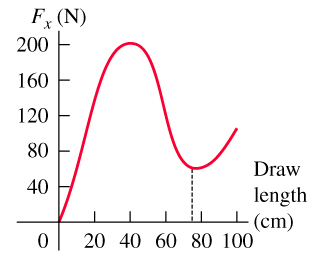# Problem: The figure shows how the force exerted by the string of a compound bow on an arrow varies as a function of how far back the arrow is pulled (the draw length). Assume that the same force is exerted on the arrow as it moves forward after being released. Full draw for this bow is at a draw length of 75.0 cm.If the bow shoots a 0.0250-kg arrow from full draw, what is the speed of the arrow as it leaves the bow?

⚠️Our tutors found the solution shown to be helpful for the problem you're searching for. We don't have the exact solution yet.

###### Problem Details

The figureshows how the force exerted by the string of a compound bow on an arrow varies as a function of how far back the arrow is pulled (the draw length). Assume that the same force is exerted on the arrow as it moves forward after being released. Full draw for this bow is at a draw length of 75.0 cm.

If the bow shoots a 0.0250-kg arrow from full draw, what is the speed of the arrow as it leaves the bow?